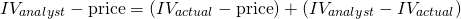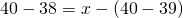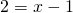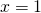# Analyst Perception of Mispricing

An analyst’s perception of mispricing is a useful framework to think about mispricing in financial markets. In particular, the analyst perception of mispricing can be decomposed into two distinct parts. One the one hand, there’s the actual mispricing, on the other hand there’s the valuation error.On this page, we discuss these two components of a perceived mispricing in more detail. It is very important for analysts to understand that, whenever they value a security, the mispricing they identify has two components.

## Analyst Perception of Mispricing formula

Let’s start with the formula. We can decompose the perceived mispricing in in the following wayIn other words, the perceived mispricing can be decomposed into the actual mispricing and a valuation error. The valuation error is the difference between the analyst’s estimate of the value of a security and the actual intrinsic value. This component will typically be at play because a valuation estimate is never exactly correct. Instead, it is accompanied by considerably uncertainty. The second component is the actual mispricing. This is the difference between the market price and the actual intrinsic value. This is the mispricing that the analyst is trying to determine.

Thus, the smaller the analyst’s valuation error, the closer the perceived mispricing will be to the actual mispricing.

## Analyst perception of mispricing example

We can illustrate the above approach using a simple numerical example. Suppose the analyst estimates that a security should trade a \$40 but it only trades at \$38. Thus the security appears to be oversold. Suppose the actual intrinsic value is \$39Thus, the actual mispricing mispricing is only \$1. Even though the analysts is correct, the security is mispricing, he nevertheless still made a valuation error

## Summary

We discussed the mispricing perceived by the analyst and decomposed it into two components; an actual mispricing and a valuation error.